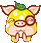登录 注册# 合成思路详解

 合成思路详解： 　　由于大家已经普遍2转，就以2转双抗性为示例。 　　No.1 　　封印 30+40+13+12 ==95 　　混乱 30+40+13+12 =95 　　遗忘 +40+13+13+13+13 =92 　　仙法 +41+5+5+5 =56 　　炼妖次数11 　　三抗 人6管制 第一种万金油打法 比较能站 (适合类型站位型血宠 常规血宠 敏宠 ) 　　点评：三个均为上限的抗性，61的仙法抗性简直硬到极致。可惜三个遗忘14确实比较土豪。 　　No.2 　　混乱 30+34+10+10+10 =94 　　封印 30+34+10+10+10 =94 　　遗忘 +34 　　物理 +39+12+12+11+11 =85 　　震慑 +19.5 　　炼妖次数10 　　5坐管制 高血量反震(适合负敏挨揍型 克制大炮 ) 　　点评：中规中矩最后一颗自由发挥，打造物理是防止物理系敏宠强行毁灭保证大炮存活。 　　No.3 　　封印 30+40+13+12 =95 　　混乱 30+40+13+12 =95 　　遗忘 +40+11+11+11+11+11 =95 　　仙法 +41+5+5 =51 　　炼妖次数11 　　三抗 人6管制 第二种万金油打法 (适合类型站位型血宠 常规血宠 敏宠 ) 　　点评：并没有上面那个硬朗，但是价格与抗性真心便宜啊~~233333 　　No.4 　　混乱 30+34+11+10+10 =95 　　封印 30+34+11+10+10 =95 　　遗忘 +34+12+12+12+12+13 =95 　　仙法 +33 　　炼妖次数11 　　三抗 五坐心如止水 换 天神护体 (适合无人6坐常规血宠打法) 　　点评：这个适合喜欢仙6 魔6 鬼6的朋友，三抗也是轻轻松松 就是这个抗仙法有点差强人意啊。 　　No.5 　　混乱30+40+12+13 =95 　　封印30+40 　　仙法+41+5+5+5+5+5+5+5+5+4 =85 　　炼妖次数：11 　　仙法抗到极致并且抗混/冰/忘均可。(适合比较主流的敏BB) 　　点评：其实这是抗仙法的敏BB打法，但是不足点显而易见极容易被控制。 　　※以上均只为示例，按照各服务器物价，可以斟酌调换，如天生抗性改成混与忘 买更多便宜的抗冰炼妖。 　　※仙法是指四系仙法。前面带“+”号的数字均是坐骑或者炼妖石加成的数字。其中，天生抗性为黑色，坐骑加成为蓝色，炼妖石为红色 　　※抗混乱炼妖石为 灵犀角 抗封印炼妖石为 云罗帐 抗遗忘炼妖石为 忆梦符 单个最高值为14(合成最高为13). 抗仙法炼妖石为 盘古石。，单个最高值为 6 (合成最高为5) <<<点击进入大话西游2论坛讨论>>> [编辑：韦小贝]

dh2.yzz.cn/guide/201507-958454.shtml

dh2.yzz.cn/jiaodian/201507-953973.shtml

dh2.yzz.cn/picture/201506-951141.shtml

dh2.yzz.cn/picture/201506-949773.shtml

dh2.yzz.cn/guide/201506-949337.shtml

dh2.yzz.cn/data/201506-947116.shtml